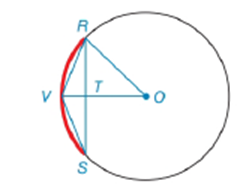Chapter 6.3, Problem 3EElementary Geometry For College St...

7th Edition
Alexander + 2 others
ISBN: 9781337614085

Solutions

Chapter
SectionElementary Geometry For College St...

7th Edition
Alexander + 2 others
ISBN: 9781337614085
Textbook Problem

Note: Exercises preceded by an asterisk are of a more challenging nature.Given: O V ¯ ⊥ R S ¯     i n     ⊙ O O V = 9     a n d     O T = 6 Find: R STo determine

To find:

To find RS.

Explanation

Given that OV¯RS¯ in O,OV=9 and OT=6

Consider the following figure,

Given that OV¯RS¯ in O then ΔORT is right triangle.

Pythagorean theorem:

In a right angled triangle then the square of the hypotenuse is equal to the sum of the squares of the other two sides.

Also OR=OV since radius are same for all points on a circle.

Consider the ΔORT to get the following,

OR2=OT2+TR2

Substitute the value of OV=OR=9 and OT=6 to get the following,

Still sussing out bartleby?

Check out a sample textbook solution.

See a sample solution

The Solution to Your Study Problems

Bartleby provides explanations to thousands of textbook problems written by our experts, many with advanced degrees!

Get Started

Evaluate each expression: 12918648+7

Elementary Technical Mathematics

In Exercises 5-8, graph the given function or equation. 2x3y=12

Finite Mathematics and Applied Calculus (MindTap Course List)

True or False: If , then converge absolutely.

Study Guide for Stewart's Single Variable Calculus: Early Transcendentals, 8th